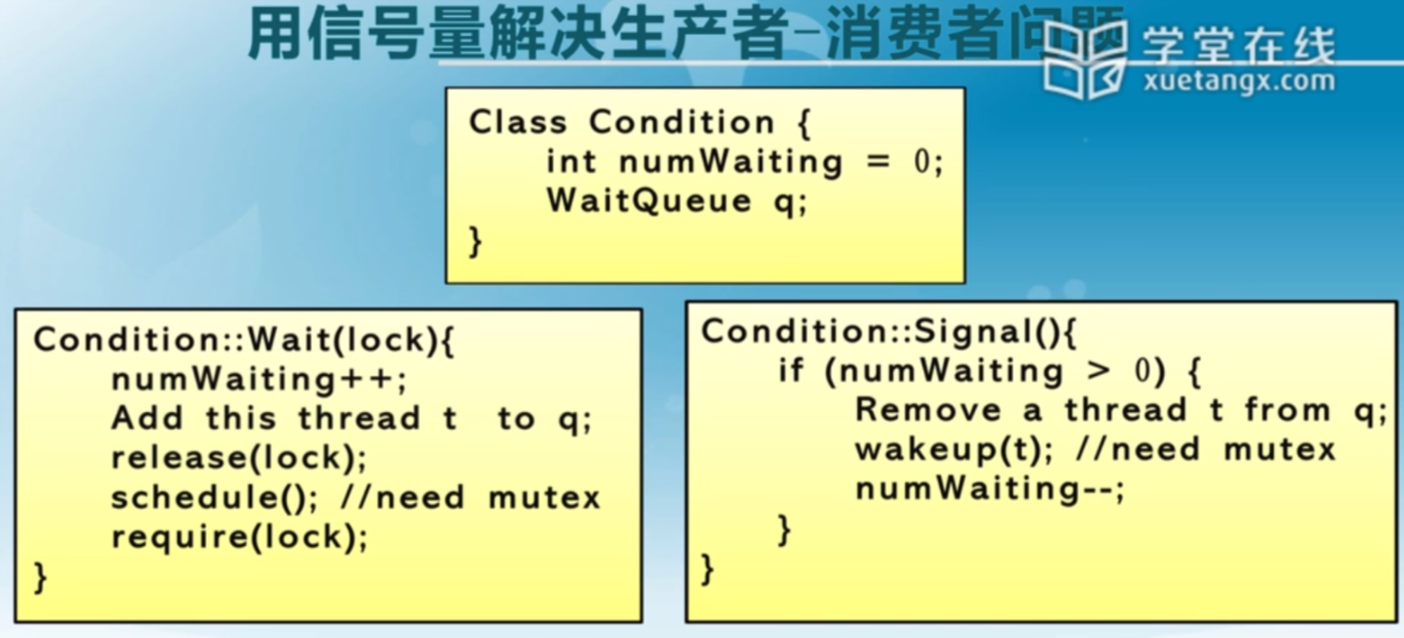2019-02-28
0 评论 0 浏览

## Process Synchronization 1

• IPC(Interprocess communication)
协作的进程需要IPC

### 三种IPC模型

• shared memory 共享内存
• pipe 管道
• message passing 消息传递

### Message passing

• 进程必须互相知道对方名字
• send(P,message)

#### 直接通信，非对称寻址

• 发送时指明了名字，接收只有id
• send(P,message)
例如：共享打印机

#### 间接通信

• 消息从中介取，往中介发 mailbox or ports
• send(A,message)

### IPC synchronization

• Blocking send ：直到上一个消息被接收时才会发下一个消息
• Non-blocking send ：随时发

### IPC buffering

• Zero capacity 队列长度=0
• Bounded capacity 队列长度=n
• Unbounded capacity 队列长度=$\infty$

### Critical-Section Problem 临界区问题

#### Race condition 竞争条件

• 如果一个进程正在使用一个共享文件或变量，其他进程不能做同样的事

#### The critical-section problem

do{
entry section   //执行前判断
crical section
exit section    //执行完毕后解锁
reminder section
}


• Mutual Exclusion 互斥
• Progress 前进原则
如果没有临界区代码在执行，有一个进程想进入临界区是，应当允许
• Bounded wait 有限等待
想进入临界区的在有限时间内总能进入

#### 例 火车问题

##### 算法1 交替进入
int turn;
turn=i  //Pi可以进入


Pi

do {
while(turn!=i);
/* critical section */
turn=j;
/* remainder section */
} while(1);


##### 算法2 flag each process 给每个进程都标记
boolean flag;
flag[i]=true;


Pi

do {
flag[i]=true;
while(flag[j]); //空循环等待j
/* critical sectioon */
flag[i]=false;
/* remainder */
} while(1);


##### 算法3 1和2的组合
do {
flag[i]=true;
turn=j; //先把机会让给另外一个
while(flag[j] && turn==j);  //如果另外一个也到了，就空循环等待
/* critical section */
flag[i]=false;
/* remainder section */
} while(1);


## Process Synchronization 2

### Synchronization tools for IPC

• Synchronization hardware
• Semaphores
• Critical Regions
• Monitors

### Synchronization hardware 硬件同步

• 单处理器可以禁止中断，使当前运行代码不会被抢占
• 在多处理器上上述方案效率太低

• 检查和修改字的内容
• 或交换两个字的内容

#### TestAndSet Instruction 检查和修改指令 自旋锁

boolean TestAndSet(boolean *target) {
boolean rv=*target;
*target=true;
return rv;
}


boolean lock=false;


do{
while(TestAndSet(&lock));
//critical section
lock=false;
//remainder section
}


#### Swap Instruction

void Swap(boolean *a,boolean *b) {
boolean temp=*a;
*a=*b;
*b=temp;
}


boolean lock=false;


do {
key=true;
while(key==true)
Swap(&lock, &key);
//critical section
lock=false;
//remainder
}


### Semaphores 信号量

Dijkstra提出

• wait()
• signal()

wait(s):

while(s<=0)
;   //no-operation
s--;


signal(s):

s++


int matex=1;


Critical section of n processes

do {
wait(mutex);
//critical section
signal(mutex);
//remainder section
} while(1);


#### 两种类型的信号量

• 计数信号量的值域不受限制，用来表示可用资源的数量
• 二进制信号量的值只能为0或1

#### 信号量实现的分析

##### 解决方法

typedef struct {
int value;
struct process *List;
} semaphore;

• Block operation: block()
• Wakeup operation: wakeup()

wait(semaphore *S){
S.value--;
if(S.value<0) {
block();    //add this process to S.List
}
}

signal(semaphore *S) {
S.value++;
if(S.value<=0) {
wakeup(P);  //remove a process P from S.List
}
}


Bounded-Buffer Problem 可用计数信号量来解决

• Starvation: 无限期阻塞

### Monitor 管程

mutex // 一个互斥锁，任何线程访问都必须先获得mutex

condition //一个或者多个，每个条件变量都包含一个等待队列

balabala //共享变量及其访问方法


#### 特点

• 采用面向对象方法，简化线程同步
• 同一时刻仅有一个线程在管程中工作
• 可临时放弃管程的访问权，叫醒一个在等待队列中的线程，这是其他方法都没有的(原子锁和信号量一旦进入临界区就必须执行完临界区代码才能退出)，而实现这一点采用的就是条件变量

• Hansan管程
• Hoare管程

#### 实现

monitor monitor-name {
shared variable declarations
procedure body P1() {   }
procedure body Pn() {   }
{initialization code}
}


#### 条件变量

• x.wait() 进程挂起
• x.signal() 被挂起的进程重新活动## Process Synchronization 3

### 1. 读写者问题

#### 前提条件

1. 写者、读者互斥访问文件资源。
2. 多个读者可以同时访问文件资源。
3. 只允许一个写者访问文件资源。

#### 两种情况

##### 1. 读者优先
• 没有读者会因为有一个写者在等待而去等待其他读者的完成
• 写者执行写操作前，应该让所有读者和写者退出
• 除非有一个写者在访问临界区，其他情况下，读者不应该等待

#### 读者优先的实现

BINARY_SEMAPHORE wrt=1;
BINARY_SEMAPHORE mutex=1;


do {
wait(mutex);    //Allow 1 reader in entry
signal(mutex);
wait(mutex);
signal(mutex);
}


Writer:

do {
wait(wrt);
//writing operation
signal(wrt);
}


#### 写者优先的实现

BINARY_SEMAPHORE read=1;    //使有写者进行操作时读者等待
BINARY_SEMAPHORE file=1;    //使文件操作互斥
BINARY_SEMAPHORE mutex2=1;  //使改变writecount的方法互斥
int writecount=0;


do {
wait(mutex1);
wait(file);     //后来的写者无法操作文件，但对后来的读者的文件操作不造成影响
signal(mutex1);
wait(mutex1);
signal(file);
signal(mutex1);
}


Writer:

do {
wait(mutex2);
writecount++;
if(writecount==1)   //第一个写者进入
signal(mutex2);
wait(file);
//write operation
signal(file);
wait(mutex2);
writecount--;
if(writecount==0)   //最后一个写者退出
signal(mutex2);
}


### 哲学家进餐问题

#### 前提条件

5个哲学家围着桌子吃饭，筷子只有5枝，分别在每个哲学家的左手边和右手边。哲学家必须得到两只筷子才能吃饭，要使每个哲学家都能吃到饭，该如何调度

#### 管程实现

void philosopher(int i) {   //主方法
while(true) {
thinking();
dp.pickup(i);
eating();
dp.putdown(i);
}
}

monitor dp {
enum{thinking,hungry,eating} state;
condition self;
void pickup(int i) {
state[i]=hungry;    //设置本人为饥饿状态
test[i];    //测试左右两个人是否在吃
if(state[i]!=eating)    //如果需要等待
self[i].wait();     //进入等待，在pickup方法处阻塞
}
void putdown(int i) {
state[i]=thinking;
test((i+4)%5);
test((i+1)%5);  //本人放下筷子后，状态发生了变化，测试左右两个是否等待吃，如有，则给他们开始吃的机会（但也不一定能马上开始吃）
}
void test(int i) {
if((state[(i+4)%5]!=eating) && (state[i]==hungry) && (state[(i+1)%5]!=eating)) {    //如果左右两个人并没有在吃，而且自己处于饥饿状态
state[i]=eating;    //设置状态为eating
self[i].signal();   //释放pickup方法，在philosopher方法中下一步将执行eating操作
}
}
initializationCode() {
for(int i=0;i<5;i++)
state[i]=thinking;
}
}


### 生产者消费者问题

#### 伪代码

semaphore mutex=1; //临界区互斥信号量
semaphore empty=n;  //空闲库存空间
semaphore full=0;  //库存初始化为空


producer ()
{
while(1)
{
produce an item in nextp;  //生产数据
P(empty);  //获取空闲库存单元
P(mutex);  //进入临界区.
V(mutex);  //离开临界区,释放互斥信号量
V(full);  //库存数加1
}
}


consumer ()
{
while(1)
{
P(full);  //获取库存数单元
P(mutex);  // 进入临界区
remove an item from buffer;  //从库存中取出数据
V (mutex);  //离开临界区，释放互斥信号量
V (empty) ;  //空闲库存数加1
consume the item;  //消费数据
}
}